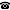# 2016 seminar talk: The tree property and the continuum function below $\aleph_\omega$

Talk held by Radek Honzík (Charles University in Prague, Czech Republic) at the KGRC seminar on 2016-04-07.

### Abstract

We say that a regular cardinal $\kappa \ge \omega$ has the tree property if there are no $\kappa$‑Aronszajn trees. It is known that if $2^\omega = \omega_1$, there are $\omega_2$‑Aronszajn trees; thus the tree property at $\omega_2$ implies the negation of CH (and analogously for larger cardinals). All the usual forcings for the tree property at $\omega_2$, such as the Mitchell forcing or the Sacks forcing, give $2^\omega = \omega_2$. We show that the “gap two” is no consequence of the tree property: indeed, we show that – starting with infinitely many weakly compact cardinals – the tree property can hold at every even cardinal below $\aleph_\omega$ and the continuum function below $\aleph_\omega$ can be arbitrary (such that $2^{\omega_2n} \ge \omega_{2n+2}, n \lt \omega$). We prove a similar result for the weak tree property as well ($\kappa$ has the weak tree property if there are no special $\kappa$‑Aronszajn trees).

This work is joint with S. Stejskalova.

Kurt Gödel Research Center for Mathematical Logic. Währinger Straße 25, 1090 Wien, Austria.+43-1-4277-50501. Last updated: 2010-12-16, 04:37.